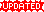# Unit 02: DifferentiationNotes (Solutions) of Unit 02: Differentiation, Calculus and Analytic Geometry, MATHEMATICS 12 (Mathematics FSc Part 2 or HSSC-II), Punjab Text Book Board Lahore. You can view online or download PDF. To view PDF, you must have PDF Reader installed on your system and it can be downloaded from Software section.

Here are few online resource, which are very helpful to find derivative.

• Introduction
• Average Rate of Change
• Derivative of a Function
• Finding $f'(x)$ from Definition of Derivative
• Derivative of $x^n$ where $n \in \mathbb{Z}$
• Exercise 2.1
• Differentiation of Expressions of the types
• Exercise 2.2
• Theorems on Differentiation
• Exercise 2.3
• The Chain Rule
• Derivatives of Inverse Function
• Derivative of a Function given in form of parametric Equations
• Differentiation of Implicit Relation
• Exercise 2.4
• Derivatives of Trigonometric Function
• Derivatives of Inverse Trigonometric Functions
• Exercise 2..5
• Derivative of Exponential Functions
• Derivative of the Logarithmic Function
• Logarithmic Differentiation
• Derivative of Hyperbolic Function
• Derivative of the Inverse Hyperbolic Function
• Exercise 2.6
• Successive Differentiation ( or Derivatives)
• Exercise 2.7
• Series Expansions of Function
• Tailor Series Expansions of Function
• Exercise 2.8
• Geometrical Interpretation of a Derivative
• Increasing and Decreasing Function
• Relative Extrema
• Critical Values and Critical Points
• Exercise 2.9
• Exercise 2.10

In this chapter many questions can be solved in much easier way. Actually in every exercise some formula/method is introduced to solve the question. In examination it is not necessary to do the same method as given in exercise. Here is one example:

We have to find the derivative of $\frac{x+1}{x-1}$ with respect to $x$.

#### Method 1

\begin{aligned} \frac{d}{dx}\left(\frac{x+1}{x-1}\right) &= \frac{(x-1)\frac{d}{dx}(x+1)-(x+1)\frac{d}{dx}(x-1)}{(x-1)^2}\\ &= \frac{(x-1)(1)-(x+1)(1)}{(x-1)^2}\\ &= \frac{x-1-x-1}{(x-1)^2}\\ &= \frac{-2}{(x-1)^2} \end{aligned}

#### Method 2

By converting improper to proper fraction $$\frac{x+1}{x-1}= 1+\frac{2}{x-1}=1+2(x-1)^{-1}$$ Now \begin{aligned} \frac{d}{dx}\left(\frac{x+1}{x-1}\right) &=\frac{d}{dx}\left(1+2(x-1)^{-1}\right)\\ &= 0-2(x-1)^{-2}(1)\\ &= \frac{-2}{(x-1)^2} \end{aligned}

This was a simple example but try it to find the derivative of $\frac{x^2+1}{x^2-1}$.

Finding a derivative is not a difficult thing. It has few rules and formulas. Instead of learning sums try to understand the rules for differentiation. Also remember derivative of important function. To remember the derivative just take a print of Important Derivatives & Integrals. At the time of solving question, use this page to see formulas. This method will help to put these formulas in your mind without any trouble.
• Exercise 2.4 | View Online | Download PDF (175KB)• Exercise 2.5 | View Online | Download PDF (239KB)• fsc/fsc_part_2_solutions/ch02